## Brainteaser: Relationship between mass and orbital distance

Posted: August 10, 2011 by tallbloke in Astronomy, Astrophysics, Solar physics, solar system dynamics

Wikipedia says:
Small body orbiting a central body
In astrodynamics the orbital period(in seconds) of a small body orbiting a central body in a circular or elliptic orbit is:where:

•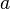is length of orbit’s semi-major axis,
•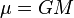is the standard gravitational parameter,
•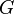is the gravitational constant,
•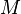the mass of the central body.

Note that for all ellipses with a given semi-major axis the orbital period is the same, regardless of eccentricity.

Orbital period as a function of central body’s density

For the Earth (and any other spherically symmetric body with the same average density) as central body we get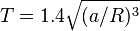and for a body of waterT in hours, with R the radius of the body.

Thus, as an alternative for using a very small number like G, the strength of universal gravity can be described using some reference material, like water: the orbital period for an orbit just above the surface of a spherical body of water is 3 hours and 18 minutes. Conversely, this can be used as a kind of “universal” unit of time.

For the Sun as central body we simply get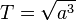T in years, with a in astronomical units. This is the same as Kepler’s Third Law
Which is that the square of the orbital period is proportional to the cube of the distance from the Sun,

Two bodies orbiting each other

In celestial mechanics when both orbiting bodies’ masses have to be taken into account the orbital period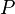can be calculated as follows: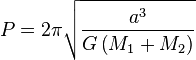where:

•is the sum of the semi-major axes of the ellipses in which the centers of the bodies move, or equivalently, the semi-major axis of the ellipse in which one body moves, in the frame of reference with the other body at the origin (which is equal to their constant separation for circular orbits),
•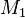and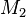are the masses of the bodies,
•is the gravitational constant.

Note that the orbital period is independent of size: for a scale model it would be the same, when densities are the same (see also Orbit#Scaling in gravity).

Wikipedia also says:

Newton’s theorem of revolving orbits

As noted by astrophysicist Subrahmanyan Chandrasekhar in his 1995 commentary on Newton’s Principia, this theorem remained largely unknown and undeveloped for over three centuries.

Consider a particle moving under an arbitrary central force F1(r) whose magnitude depends only on the distance r between the particle and a fixed center. Since the motion of a particle under a central force always lies in a plane, the position of the particle can be described by polar coordinates (rθ1), the radius and angle of the particle relative to the center of force (Figure 1). Both of these coordinates, r(t) and θ1(t), change with time t as the particle moves.

Imagine a second particle with the same mass m and with the same radial motion r(t), but one whose angular speed is k times faster than that of the first particle, where k is any constant. In other words, the azimuthal angles of the two particles are related by the equation θ2(t) = k θ1(t). Newton showed that the motion of the second particle can be produced by adding an inverse-cube central force to whatever force F1(r) acts on the first particlewhere L1 is the magnitude of the first particle’s angular momentum, which is a constant of motion (conserved) for central forces.

If k2 is greater than one, F2 − F1 is a negative number; thus, the added inverse-cube force is attractive, as observed in the green planet of Figures 1–4 and 9. By contrast, if k2 is less than one, F2F1 is a positive number; the added inverse-cube force is repulsive, as observed in the green planet of Figures 5 and 10, and in the red planet of Figures 4 and 5.

and this:

Two types of central forces—those that increase linearly with distance, F = Cr, such as Hooke’s law, and inverse-square forces, F = C/r2, such as Newton’s law of universal gravitation and Coulomb’s law—have a very unusual property. A particle moving under either type of force always returns to its starting place with its initial velocity, provided that it lacks sufficient energy to move out to infinity. In other words, the path of a bound particle is always closed and its motion repeats indefinitely, no matter what its initial position or velocity. As shown by Bertrand’s theorem, this property is not true for other types of forces; in general, a particle will not return to its starting point with the same velocity.

However, Newton’s theorem shows that an inverse-cubic force may be applied to a particle moving under a linear or inverse-square force such that its orbit remains closed, provided that k equals a rational number. (A number is called “rational” if it can be written as a fraction m/n, where m and n are integers.) In such cases, the addition of the inverse-cubic force causes the particle to complete m rotations about the center of force in the same time that the original particle completes n rotations. This method for producing closed orbits does not violate Bertrand’s theorem, because the added inverse-cubic force depends on the initial velocity of the particle.

Harmonic and subharmonic orbits are special types of such closed orbits. A closed trajectory is called a harmonic orbit if k is an integer, i.e., if n = 1 in the formula k = m/n. For example, if k = 3 (green planet in Figures 1 and 4, green orbit in Figure 9), the resulting orbit is the third harmonic of the original orbit. Conversely, the closed trajectory is called a subharmonic orbit if k is the inverse of an integer, i.e., if m = 1 in the formula k = m/n. For example, if k = 1/3 (green planet in Figure 5, green orbit in Figure 10), the resulting orbit is called the third subharmonic of the original orbit. Although such orbits are unlikely to occur in nature, they are helpful for illustrating Newton’s theorem.

———————————————–

All of which is very interesting, Newtons thoughts on inverse cube forces put me in mind of electromagnetic forces which wax and wane on a period matching the Jupiter-Saturn synodic cycle for example. This is observed in the spectral analysis of the sunspot record, which correlates loosely with solar wind density and speed. Recalling P.G.’s comment on the previous thread about the charge carried by planets and the Sun, we might consider an inverse cube force acting not only between the sun and, e.g.  Jupiter, but also between Jupiter and Saturn. Which puts us in mind of Miles Mathis’ gravitational theory, of an attractive force slightly stronger than G and a repulsive force which reduces that force to G in the frame of reference we are familiar with: Terrestrial gravitational effects.

Although the orbits of Jupiter and Saturn would be unaffected on average, we might expect the purturbation over the synodic cycles to help maintain that whole integer K factor which in turn keeps the planets in synch with solar activity. This would go for Earth, Venus and Mercury too, as Ulric points out on the ‘Jackpot’ thread. The 5-8 orbit coupling between Earth and Venus being especially noticeable, but distracting us from Mercury’s contribution to solar flare discharge triggering (Ching Cheh Hung).

There’s plenty to think about as we integrate classical celestial mechanics with some up to date results and observations which point to a strong electromagnetic element in the tuning of the solar system rhythms.

Comments
1.tchannon says:

Mass and orbital distance?

2.Tenuc says:

Roger, according to Miles, gravity is an acceleration (measured in m/s^2), not a force and that the only force involved comes from the charge field which is in direct opposition to the apparent pull of gravity for a 2 body system. This force from the charge field is caused by bombardment photons which have both mass and size, and which deliver real kinetic energy to the bodies involved. The PE caused by the acceleration of gravity is only expressed when two objects collide.

3.tallbloke says:

Lol. 🙂

Mind the store Tim, I’m away for a few days.

Cheers.

4.tallbloke says:

Roger, according to Miles, gravity is an acceleration (measured in m/s^2), not a force

Tenuc, quite so. As I understand it, Miles says the acceleration and apparent ‘pull’ are equivalent, apart from the E/M component.

The PE caused by the acceleration of gravity is only expressed when two objects collide.

Falling is easy. It’s landing which causes the problem. 🙂

5.Willis Eschenbach says:

tallbloke, interesting post. However, you say:

This is observed in the spectral analysis of the sunspot record, which correlates loosely with solar wind density and speed.

I’m sure it does. It also likely correlates loosely with a whole host of phenomena.

But since you are not making the point that there is a statistically significant correlation with solar wind density, I’m not sure what your point is.

This is a recurring problem with cyclical analyses. Yes, there are all kinds of loose correlations. There are dozens and dozens of cycles in even a simple three-body problem like the oceanic tides. There are no less than 51! sun/earth/moon cycles that are used in that calculation. Fifty one different cycles, and you know what?

One or more of the fifty-one tidal components likely “correlate loosely” with some other cyclical phenomenon …

So what? Until you can show that a) the correlation is statistically significant and b) there is a causational link between the two, all you’re doing is playing with cycles. Interesting, as I said above, but non-productive.

You’re getting caught in a statistical oddity. You look at a correlation between two things, and if you find a correlation which is statistically significant at say the p=0.05 level, you think you’ve found something.

But if to find those two things you looked at oh, say, a dozen different pairs, is your p=0.05 still significant?

Absolutely not. The odds of p=0.05 being a random occurrence if you look at 12 pairs of cycles is 1 – 0.95^12, which is about a fifty-fifty chance … and how many pairs of cycles did you look through to find the pair under discussion?

Many thanks,

w.

6.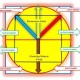adolfogiurfa says:

What, then, is mass? Is it energy just “congealed”, by the observer?. Remembered the words of Sir Arthur Eddington at a conference where he described the desk before him as “moving atoms”…

7.tallbloke says:

Hi Willis,

Oh well, no-one ever thought it was going to be easy.
Fortunately, apart from the rather enigmatic and capricious changes in the solar wind, we have some very tight and exact correlations to use as co-ordinate beacons to help us thread our way around the problem.

Your statistical observations don’t worry me much, because we have thought about the logic of the situation quite a bit. We don’t rate a correlation between, say, Jupiter and pluto synodic period and solar flares in the same way as the eight very clear correlations we find between Jupiter and Saturn (the two largest planets in the system) and the Sun’s rotation rates, sunspot count and changes in .magnetic indices.

So we are not deterred by defeatist probability reckonings. 🙂

Cheers

tb

8.Ray Tomes says:

I have looked at these matters from a slightly different but related perspective. Because the densities of bodies are not random but clustered around certain values, the orbital periods of a small body just above the surface of a planet are also concentrated around certain values. These values turn out to be ~85 minutes, ~170 minutes and ~255 minutes, or multiples of ~85 minutes. This is a clear case of a tendency to quantization. The periods of 80 and 160 minutes are also found in many other relationships.
(1) The outer planets are on the nodes of a 160 light minute wave from the Sun except Jupiter on the node of an 80 light minute wave.
(2) Binary stars have a tendency to revolve in 160 minutes or periods that commute with that (160/n or 160*n with n integer). See Kotov.
(3) Some planets rotate in periods near to k^2*160 minutes with k an integer (k=2 for Jupietr and Saturn, 3 for Earth and Mars) while others rotate in k^2*6.4 days (k=1 for Pluto, 2 for Sun, 3 for Mercury and 6 for Venus).
(4) Periods of 160 minutes have been observed in black holes at galactic cores.
(5) The known masses of black holes show a tendence to multiples and fractions of 160 minutes equivalent event horizon radius.

IMO all of this comes about because the Universe has all pervading standing waves of 160 minutes and related periods. See http://ray.tomes.biz/maths.html

9.Willis Eschenbach says:

tallbloke says:
August 10, 2011 at 8:24 pm

Hi Willis,

Oh well, no-one ever thought it was going to be easy.
Fortunately, apart from the rather enigmatic and capricious changes in the solar wind, we have some very tight and exact correlations to use as co-ordinate beacons to help us thread our way around the problem.

Your statistical observations don’t worry me much, because we have thought about the logic of the situation quite a bit. We don’t rate a correlation between, say, Jupiter and pluto synodic period and solar flares in the same way as the eight very clear correlations we find between Jupiter and Saturn (the two largest planets in the system) and the Sun’s rotation rates, sunspot count and changes in .magnetic indices.

So we are not deterred by defeatist probability reckonings.

Cheers

tb

Thanks, Rog. I understand that you are “not deterred by defeatist probability reckonings”, but you are also not supported by actual statistical calculations … which was my point.

So you can say “eight clear correlations”, but until you can establish what “clear” means in a mathematical sense, it’s just handwaving. Interesting handwaving to be sure, indeed fascinating at times … but handwaving nonetheless.

I asked before on the other thread and got no answer … but what did you predict for the current sunspot cycle, and how is your prediction working out? It’s easy as pie to find “clear correlations” in all kinds of data. It’s using them to predict the future that’s hard.

All the best,

w.

10.P.G. Sharrow says:

Willis thinks we are digging in a pile of manure.

Roger thinks that there is a pony in here somewhere.

At least the garden is growing very well! 😎 I’m happy. pg

11.steven mosher says:

Nicely put willis,

TB. Here is the point. You might not be deterred by the probablistic concerns that willis and I have. Fine. The point is this. We are rationally justified in rejecting any correlation that you find with a such a process, especially in the absence of a testable physical theory. There is nothing we have to prove “wrong” because by snooping through multiple cycles multiple times you have destroyed any statistical significance. You of course are free to continue to rummage through cycles to find matches. You will find them galore! I predict that with confidence. But until you account for the decrease in significance From multiple looks and until you have a testable equation, don’t pretend that we are somehow ignoring what you have found.

12.Richard Holle says:

What is happening on this blog is the gathering of lots of tubes of paint, (cyclic patterns) when we find the brushes (the mechanics driving the cycles based on electromagnetic data) and a good set of canvases upon which to flesh out how the mechanics are controlled by or controlling the effects, seen in the data soon to be collected as more satellite info can be accessed, the patterns will appear in a form that the mathematics will out line the proposed constructs seen in the pictures.

Science is understanding how nature works, that it does somehow is easy to see, the methods of action can be sensed, but it is hard to visualize the math directly until patterns in the data lead to mechanisms. Art is the ability to express what is felt and understood, but hard to communicate, but easily seen when finished.

13.tallbloke says:

[Waves to Willis] 🙂

Hi Willis and Mosh,

Well guys, call it rummaging, or handwaving, or whatever you like. It’s clear something is behind the ‘coincidences’ we observe so you’re welcome to join in trying to discover what is going on in the solar system, or stick with conducting your significance tests or whatever floats your boat. Your efforts to keep us on the straight and narrow are appreciated. We’ll do our best to formulate our claims correctly when we are ready to make some.

Speaking for myself, I’m happy to continue publishing the fascinating discoveries and ideas of the contributors to the talkshop, because I’m satisfied that these synchronous phenomena are not happening by chance. When we’ve found a way to predict the amplitude of shorter term solar changes (a tall order in the Sun’s currently very unpredictable phase), we’ll let you know, so you can test the accuracy of our results.

I just got back from a great music festival, so comments get accompanying listening recommendations at the moment. These guys played on Friday night, and stole the show.

14.Paul Vaughan says:

On volunteer diversity:
I think some misunderstand tallbloke’s role.
Plenty of musical hats to be worn.
Plenty of opportunity to disagree harmoniously.
No need to all play the same instrument the same way, etc…
Counterpoint can be woven tastefully.

Cheers.

15.Paul Vaughan says:

On Stat Inference:
I spent years of my life teaching the fundamentals (i.e. assumptions) of this stuff. Why defend, advocate, &/or promote a paradigm that’s inappropriate (potentially very seriously misleading) for the nature of the problem at hand? Abstract stat model assumptions are patently untenable given current levels of understanding of terrestrial climate. If proper data exploration gets underway, one day knowledge of conditioning may be sufficient to entertain meaningful inference. (Note: Data exploration & statistical inference differ fundamentally.) Stat culture dominance depends on getting algebraic wool, in the form of untenable assumptions, over potentially realistic eyes. Some can’t see past the floods of algebra. They aren’t a threat to dominance. They usually opt for obedience. A rare few with lucid awareness just “go with it”, some with a smug grin. (Again: Data exploration & statistical inference differ fundamentally.)

16.P.G. Sharrow says:

TB; After the problem is solved it will be clear to Willis and Mosh. Or maybe when it is clear to them, then the problem is solved. 😎 pg